## Wednesday, July 15, 2009

### Data Preprocessing – Normalization

Further to introduction, in this article I am going to discuss “Data Preprocessing” an important step in the knowledge discovery process, can be even considered as a fundamental building block of data mining. People who come from data warehousing background may already be familiar with the term ETL ( Stands for Extraction,Transformation and Loading). Any data mining or data warehousing effort's success is dependent on how good the ETL is performed. DP ( I am going to refer Data preprocessing as DP henceforth) is a part of ETL, its nothing but transforming the data. To be more precise modifying the source data in to a different format which
(i) enables data mining algorithms to be applied easily
(ii) improves the effectiveness and the performance of the mining algorithms
(iii) represents the data in easily understandable format for both humans and machines
(iv) supports faster data retrieval from databases
(v) makes the data suitable for a specific analysis to be performed.

The real world data is mere junk without DP. I am going to explain this through a simple example based on Normalization. For people who come from database background this Normalization is completely different from 1st, 2nd and 3rd form of normalizations used in the relational database design. This is a data preprocessing technique, You are going to say Wow !! to see how a simple DP technique in action can improve the effectiveness of analysis in orders of magnitude. To move on further we should know what is euclidean distance ?

Consider two points in a two-dimensional space (x1,y1) and (x2,y2), the distance between
these 2 points is given by the formula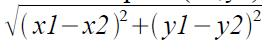This is called the Euclidean distance between the points. The same concept can be extended easily to multidimensional space. If the points are (a1,b1,c1,d1...) and (a2,b2,c2,d2,...), the distance between the points is given by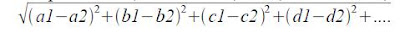Now, I am going to introduce a sample data set of employee details. The assignment is to find the employees who have similar profiles.

 Empid Salary Age Experience 1 25000 24 4 2 40000 27 5 3 55000 32 7 4 27000 25 5 5 53000 30 5

In this data set the first column is an identifier and the rest of the columns contain information. Ignoring the id column if we consider each column as a dimension we can assume that each employee is represented by a point in three dimensional space. The good thing is we can use the euclidean distance formula to calculate the distance between each of these points. The distance between the points 1 and 2, ( the Emp ids 1 and 2 ) can be calculated as below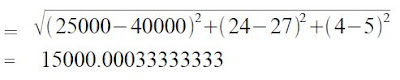Look at the data set above, through examination we find that emp id 1 and 4 are employees with almost similar profile. So in the three dimensional space the distance between them should be less, or to put it other way these two points should be very close.

The following table gives the euclidean distance calculation for each employee with the other employee in our example data set.

 1 2 3 4 5 1 0 15000 30000 2000 28000 2 15000 0 15000 13000 13000 3 30000 15000 0 28000 2000 4 2000 13000 28000 0 26000 5 28000 13000 2000 26000 0

we can see from the above list that the distance between the employee no 1 and 4 is 2000.0004999999376, which is less when compared to distance between Empid 1 and other employees.So our interpretation seems to be correct. An alert reader would have noticed a significant thing here. Wait a minute and observe the above list again, it looks like the euclidean distance values are very close to the differences in salaries.

See this, the difference between the salaries of employees 1 and 4 is
= abs(25000 – 27000) = 2000

And the euclidean distance between one and four is 2000.0004999999376. Oh God !! The approach seems to be flawed. The euclidean distances are dominated by salary amount. So guess you cant rely on euclidean distance to find employees with similar profile.

How do we get ourselves out of this problem? We do not want the salary amount to dominate the euclidean distance calculation. We can achieve this by applying the DP technique, Normalization over the example data set.

What is Normalization?
The attribute data is scaled to fit in a specific range. There are many type of normalization
available, we will see one technique called Min Max Normalization here. Min Max Normalization transforms a value A to B which fits in the range[C,D]. It is given my the below formula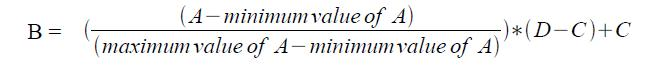Consider the below example, the salary value is 50000, we want to transform this in to the
range [0.0, 1.0], the maximum value of salary is 55000 and the minimum value of salary is 25000 so the new scaled value for 50000 will be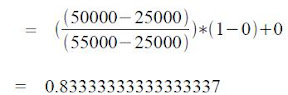Now let us apply the this normalization technique to all the three attributes in our example
dataset. Consider the following maximum and minimum values

Max salary = 55001
Min Salary = 24999
Max age = 33
Min age = 23
Max experience = 8
Min experience = 3

The attributes need to scaled to fit in the range [0.0,1.0]. Applying the min max
normalization formula above, we get the normalised example data set as given below

Normalised Example dataset

 Empid Salary Age Experience 1 0.0000333 0.1000000 0.2000000 2 0.5000000 0.4000000 0.4000000 3 1.0000000 0.9000000 0.8000000 4 0.0700000 0.2000000 0.4000000 5 0.9300000 0.7000000 0.4000000

Actual Employee data set before normalization ( to make the comparison easier)

 Empid Salary Age Experience 1 25000 24 4 2 40000 27 5 3 55000 32 7 4 27000 25 5 5 53000 30 5

We will calculate the euclidean distances for each employee with the other employee now. Its given in the table below.

Euclidean distance after normalization

 1 2 3 4 5 1 0 0.616414 1.41417 0.233332 1.12738 2 0.616414 0 0.812383 0.477235 0.527022 3 1.41417 0.812383 0 1.23329 0.452155 4 0.233332 0.477235 1.23329 0 1.00051 5 1.12738 0.527022 0.452155 1.00051 0

Euclidean distance before normalization

 1 2 3 4 5 1 0 15000 30000 2000 28000 2 15000 0 15000 13000 13000 3 30000 15000 0 28000 2000 4 2000 13000 28000 0 26000 5 28000 13000 2000 26000 0

Now compare the euclidean distance calculation pre and post normalization. We can see that the distances are no more dominated by the salary amount and they make more sense now. The age and the experience also contribute to the distance calculation. Its amazing !! Isn't it? I told you, right in the beginning you will get a Wow!! experience to see DP in action.

To conclude there are many measures similar to euclidean distance which can be used to calculate the similarity between two records such as Pearson coefficient, Tanimoto Coefficient, you can try replacing euclidean distance with any of these measures and experiment. You can read more on these measures in the see also section of the link and more on other normalization techniques such as X^2 ( X square) Normalization and decimal scaling are also worth trying. would appreciate your comments and gifts ;)

1.Looks like a Maths Session :-)

2.A good blog on data processing and normalization..Quiet an interesting approach using the mathematical terms and methods...
Need a little patience to go through the mathematical calculations :)

3.Quick question:
- What would you do if your actual Employee data set before normalization was instead;

Empid Salary Age Experience
1 25,000 24 4
2 40,000 27 5
3 5,550,000 32 7
4 27,000 25 5
5 53,000 30 5

Lets assume all the data is correct (the outlier is a valid value).

4.In Reply to Anonymous question

I appreciate that its a brilliant question.

Outliers can be found by using a technique called box plot (http://en.wikipedia.org/wiki/Box_plot)

Before we normalize the data set, we need to check for outliers

There are two possible ways to handle outliers in the data
1. Ignore them, remove from the data set (or)
2. Reassign the value to of appropriate upper or lower threshold.

Thanks,
Venki

5.good post. Expecting more mathematic related data mining article regularly.

6.Great post, thank you for clarifying the role of normalization. Is there a way to normalize non-numeric values? Like "little", "big", "huge"?

7.@Dave,
To use non numeric labels such as little,big and huge we may first need to convert them in to numerical values.
Say for eg. If i refer the length 50-100 cms as small,
The measurements 75,85,55,70 fall in this range
to normalise the measurement attribute
(i) I can use the actual measurement values
(ii) I can consider all the above measurements equivalent to a value of upper or lower threshold, which ever is appropriate based on the analysis. In this case i can consider the measurements which fall under the range 50-100
equivalent to 100 cms

Normalization mostly makes sense only to numerical data, so we can make use of normalization only in cases where appropriate numerical representation is possible for an attribute.

Thanks,
Venki

8.Normalization as you describe will matter more to numeric solutions (regressions, neural networks, etc.) than to logical ones (tree and rule induction). In fact, most logical solutions will not be affected by this process at all.

Another interesting issue is the handling of missing values. Although some modeling procedures can deal with missing values directly, most cannot and some accommodation must be made.

9.Interessting post! Hope to see more.. :)

10.Very interesting examples. Regarding timeliness, DP is not done in my opinion during ETL. DW fields are "pre-processed" before building a particular data mining model, and therefore transformations on the same field may difer from one model to another (depending for instance on the algorithm to be used). ETL is done before, in order to populate the DW with information.

11.@ Matt,
Thanks for the comment. I am working on next post on real time examples for normalization and bayesian classifiers. Hope to publish them soon.

@Carlos
I agree with you, what you said is correct when the data mining models are built from data available from a DW. But in cases where a seperate DW entity does not exist, the transformation involved here can be considered as a part of ETL.This applies to the cases where a data mining model is rapidly built from raw data. Anyway there is no strict distinction which can be made.Thanks for the comment.

12.Thanks, it was very funny, valuable and instructional post.

13.its really good

14.Hi,
Can you explain how normalization works in the following cases.
1. You have a training set and you are implementing k-cross validation on it to evaluate k-nn classifier performance and come up with a good value of k. Do you normalize ONLY the training set in that case and then running k-nn on every test set to predict it's class? Or do you normalize ALL the test+training records before partitioning to k partitions and running tests?

2. When you finally use k-nn classifier to predict classes unknown from a final test set, do you normalize the test set on its own first and then compare using k-nn against the normalized training set to see k nearest neighbours? Or do you not normalize a test set at all?

If you could explain that then that would be awesome! :)

Cheers!

15.Let me summarize How i would approach it

1. Find the higher and lower boundary values in the training set, modify any outliers to the lower or higher boundary value appropriately ( a box plot would help for this, fix 85th percentile in the box plot as higher value and say 5th to 10th percentile as lower value). Use this boundary values for normalizing the training set.I assume that I am going for min max normalization here.

2. Get a box plot for the test data and check for outliers, whether 85th percentile of training data and test data are close at least, if there is a huge difference then basing the normalization of test data on the high and lower boundary values from the training data will not be accurate or to be simple, our training data does not contain a good representative data set.

16.excellent article ...
btw i have just one question, can we give weightage while calculating similarities/disimilarities. For example: i want to give more weightage to the age variable as it maybe a strong predictor. Could you please provide your suggestion for this scenario ?

Thanks
Deepak
http://prdeepakbabu.wordpress.com

17.this method compares one record to another single records

how about many records to single record?

say 10K customers out of 100K respondered to a marketing campaign. and now you wanted to run the campaign again to a new set of 100K customers but wanted to target those who were similar to the 10K responders.

how would you do this?

18.@petros
I would approach your problem as below

Run a self learning clustering algorithm on 10K responders and identify the clusters and build a cluster model.

Use the cluster model with the new set of 100k customers and find the people who fall in the clusters same as that of initial 10k responders.

The cluster model can be further improved based on the responders from the new set of customers.

Thats a good question !! Thanks :)

Venki

19.Excellent Article.
Brilliantly Explained.
Keep up the good work.

20."the salary value is 50000" - Is this an assumed value? how do u get this value in above min-max norm example?

21.@Talal
Hi, 50000 is just a sample salary value assumed to explain how min-max norm works.

22.do you have any programing for normalizing ? algorithm.

23.thanks ...really want such explanation :)

24.@Anum: Thanks a lot !!

25.Hello,
I've readed this entry, which seems really interesting. But I'd like to do a comment / question:
What if a new employee with age 22, 34 or even higher/lower (without being an outlier) should be compared? Min/Max values should be updated?

Thanks.

26.Excellent tutorial on Data Preprocessing.Ver well explained. "Hats off to Venki".

27.@Yogendra: Thanks for listening :)

28.thank you so much. very helpful and this is exactly what I was looking for...

29.very nice job thankyou venki

30.thanks so much Mr. Venki
this really helps my brain to understand what normalization is for :)

31.Explained nicely,,, Thanks

-- K. Raza

32.thanks it helps to understand in an easy way

33.I followed ur example for min-max normalization.
Thank you for the hard work you put in.

34.Just in case people have not realised ...

The Euclidean distance in 2 dimensions for Cartesian coordinates is simply a re-statement of Pythagoras's Law - where the distance is the hypotenuse.

It is simply extended to more dimensions by using the extra coordinates terms as given above.

35.there are some data sets.but some are missing values in solumn age.then I need to find that missing value age?how can I do this?

36.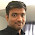@Nilu, you can predict the missing values, by considering them as labels and running a learning on examples without missing values.

37.@Venki please, can you advice me on the following:
1. the existing methods of pre-processing.(with explanations)
2. To develop a generic way of pre-processing data.
3. How to use this with different scenarios (different type of data)
4. the generic method will be in a form of algorithms.
5. can you advice me on ways of developing a generic method for pre processing data so that it can fit into any tool like neural network or linear regression. etc.

38.Thanks for your explanation! In my clustering, the datasets need to normalization for adjusting parameter value, but standard deviation value is so small.So, number of the cluster is one. So, do normalization effect the dataset nature and which normalization is suitable for what types of dataset?

39.@snow how does your clustering results look like when you do not normalize? what type of clustering and what is the distance function that you are using?

40.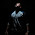Can we normelize just one attribute ?? like salary !!

1.yes sure we can. We should keep in mind that normalizing one attribute could make it significance lesser or more depending upon the chosen range.

41.This comment has been removed by the author.

42.Venkatesh,
Do you take on project work for data mining / ETL. If so I would like you to look at some files and get your impression of the scope. Thanks

1.@BillLehn, yes of course. please contact me at venkatesh20 [at] gmail [dot] com

43.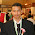Thanks, It's become simpler with your explaination

44.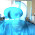Are you aware of this post http://aimotion.blogspot.com.ee/2009/09/data-mining-in-practice.html Seems like your post was first. Someone has been copying :-/